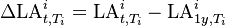# Credit PnL

## Definition

Credit PnL (Profit and Loss) denotes the delta in the measurement of the value of credit assets over a given period. This value can be computed on a mark-to-market basis or an accounting basis (e.g. under IFRS 9 / CECL)

## IFRS 9 Notation

In the context IFRS 9 we define the "PnL" on a credit asset narrowly as the change in Loss Allowance from period to period. Hence we do not mingle changes in effective interest rate or changes in the net amount accruing interest (as required by IFRS 9 for assets migrating to Stage 3). The PnL can defined and calculate for any interval, we focus on the 1y risk horizon:$\Delta \mbox{LA}_{t,T_i}^{i} = \mbox{LA}_{t,T_i}^{i} - \mbox{LA}_{1y,T_i}^{i}$